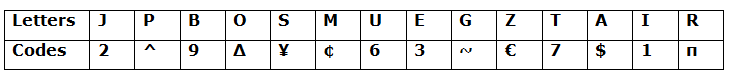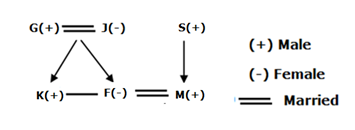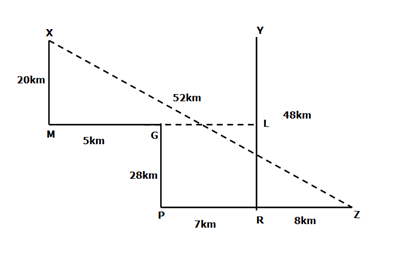# SBI PO Prelims Reasoning (Day-28)

Dear Aspirants, Our IBPS Guide team is providing new series of Reasoning Questions for SBI PO 2020 Prelims so the aspirants can practice it on a daily basis. These questions are framed by our skilled experts after understanding your needs thoroughly. Aspirants can practice these new series questions daily to familiarize with the exact exam pattern and make your preparation effective.

Start Quiz

Ensure Your Ability Before the ExamTake SBI PO 2020 Prelims Free Mock Test

Coding decoding

Direction (1-4): Study the following information carefully and answer the following question.Conditions:

I)If the 1stletter is followed by a vowel and the last letter is a consonant, the codes of both of these letters (V&C) are to be interchanged.

II)If both the 1stand the 2nd last letters are vowels then both of these letters are to be coded as €.x

III) If the 1st letter is a consonant and last letter is a vowel then both of these letters are to be coded as per the code of the last letter.

Remaining letters are coded as per the given codes.

1) EMPIUB

A.3¢^139

B.6¢1^39

C.€¢^16€

D.€¢^1€9

E.9¢^16€

2) TROGSZ

A.€π∆~¥7

B.€π∆¥~7

C.7π∆~¥€

D.7π∆~€¥

E.7π~∆¥€

3) SAGMJR

A.π¥~¢2\$

B.π\$~¢2¥

C.¥π~¢2¥

D.¥\$~¢2π

E.¥π~¢2\$

4) PTSOZI

A.17¥∆€1

B.17¥∆21

C.^7¥∆€1

D.17¥∆€7

E.^7¥∆1€

Blood relation

Direction (5-6): Study the following information carefully and answer the below questions

In a family there are six persons viz. F, J, G, K, M, and S and has two generations. K and M are brothers-in-laws. F is the only daughter of G. G and S are of same gender. F is the daughter-in-law of S who is not a parent of K. J is the mother of F.M does not have any siblings.

5) How J is related to K?

A.Mother

B.Father

C.Mother-in-law

D.Father-in-law

E.Can’t be determined

6) Who among the following persons is the Son-in-law of G?

A.The one who is the brother of F

B.S

C.K

D.The one who is the son of S

E.None of the above

Misc

7) If a four letter meaningful word can be formed by using the second, fourth, sixth and seventh letters of the word “JOURNAL” then what is the second letter from the left end of the newly formed word? Mark X as your answer if more than one word is formed. Mark Z if no meaningful word can be formed.

A.R

B.A

C.L

D.X

E.Z

Direction sense

Direction (8-10): Study the following information carefully and answer the below questions

Point X is 20km north of point M which is 5km west of point G. Point Z is 15km east of point P which is 7km west of R. Point Y is north of R and East of Point X. point P 28km south of point G.

8) What is the direction of point G with respect to point R?

A.East

B.West

C.North-west

D.South

E.South-west

9) What is the shortest distance between Points Z and X?

A.48 km

B.42 km

C.58 km

D.52 km

E.Can’t be determined

10) If Point L lies between the points Y and R and is to the east of Point G, then what is the distance between the points L and M?

A.22km

B.20km

C.15km

D.10km

E.12km

Directions (1-4) :

2) Answer: C (No Condition applies, coded as per the given codes)

4) Answer: A (Condition –> III)

Directions (5-6) :Oral

Directions (8-10):482+202=(ZX)2

√(482+202)=√2704=52km